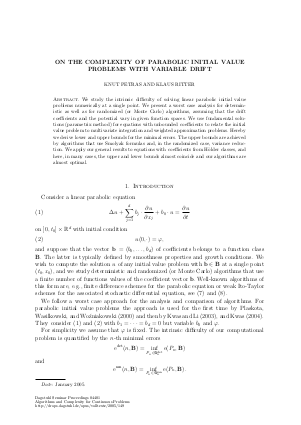Document# On the Complexity of Parabolic Initial Value Problems with Variable Drift

### Authors Knut Petras, Klaus Ritter## File

DagSemProc.04401.10.pdf
• Filesize: 297 kB
• 24 pages

## Cite As

Knut Petras and Klaus Ritter. On the Complexity of Parabolic Initial Value Problems with Variable Drift. In Algorithms and Complexity for Continuous Problems. Dagstuhl Seminar Proceedings, Volume 4401, pp. 1-24, Schloss Dagstuhl - Leibniz-Zentrum für Informatik (2005)
https://doi.org/10.4230/DagSemProc.04401.10

## Abstract

We consider linear parabolic initial value problems of second order in several dimensions. The initial condition is supposed to be fixed and we investigate the comutational complexity if the coefficients of the parabolic equations may vary in certain function spaces. Using the parametrix method (or Neumann series), we prove that lower bounds for the error of numerical methods are related to lower bounds for integration problems. On the other hand, approximating the Neumann series with Smolyak's method, we show that the problem is not much harder than a certain approximation problem. For HÃƒÂ¶lder classes on compact sets, e.g., lower and upper bounds are close together, such that we have an almost optimal method.
##### Keywords
• Partial differential equations
• parabolic problems
• Smolyak method
• optimal methods

## Metrics

• Access Statistics
• Total Accesses (updated on a weekly basis)
0